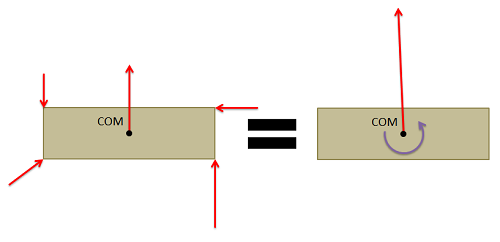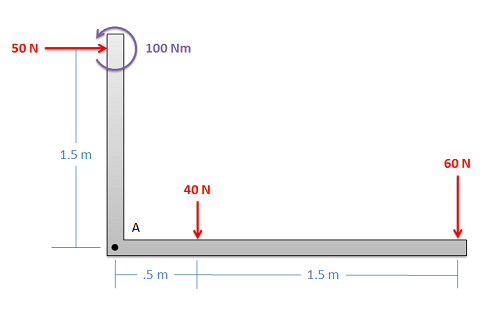# 4.3: Equivalent Force Couple System

$$\newcommand{\vecs}{\overset { \rightharpoonup} {\mathbf{#1}} }$$ $$\newcommand{\vecd}{\overset{-\!-\!\rightharpoonup}{\vphantom{a}\smash {#1}}}$$$$\newcommand{\id}{\mathrm{id}}$$ $$\newcommand{\Span}{\mathrm{span}}$$ $$\newcommand{\kernel}{\mathrm{null}\,}$$ $$\newcommand{\range}{\mathrm{range}\,}$$ $$\newcommand{\RealPart}{\mathrm{Re}}$$ $$\newcommand{\ImaginaryPart}{\mathrm{Im}}$$ $$\newcommand{\Argument}{\mathrm{Arg}}$$ $$\newcommand{\norm}{\| #1 \|}$$ $$\newcommand{\inner}{\langle #1, #2 \rangle}$$ $$\newcommand{\Span}{\mathrm{span}}$$ $$\newcommand{\id}{\mathrm{id}}$$ $$\newcommand{\Span}{\mathrm{span}}$$ $$\newcommand{\kernel}{\mathrm{null}\,}$$ $$\newcommand{\range}{\mathrm{range}\,}$$ $$\newcommand{\RealPart}{\mathrm{Re}}$$ $$\newcommand{\ImaginaryPart}{\mathrm{Im}}$$ $$\newcommand{\Argument}{\mathrm{Arg}}$$ $$\newcommand{\norm}{\| #1 \|}$$ $$\newcommand{\inner}{\langle #1, #2 \rangle}$$ $$\newcommand{\Span}{\mathrm{span}}$$$$\newcommand{\AA}{\unicode[.8,0]{x212B}}$$

Every set of forces and moments has an equivalent force couple system. This is a single force and pure moment (couple) acting at a single point that is statically equivalent to the original set of forces and moments.Figure $$\PageIndex{1}$$: Any set of forces on a body can be replaced by a single force and a single couple acting that is statically equivalent to the original set of forces and moments. This set of an equivalent force and a couple is known as the equivalent force couple system.

To find the equivalent force couple system, you simply need to follow the steps below.

1. First, choose a point to take the equivalent force couple system about. Any point will work, but the point you choose will affect the final values you find for the equivalent force couple system. Traditionally, this point will either be the center of mass of the body or some connection point for the body.
2. Next, resolve all the forces not acting though that point to a force and a couple acting at the point you chose.
3. To find the "force" part of the equivalent force couple system, add together all the force vectors. This will give you the magnitude and the direction of the force in the equivalent force couple system.
4. To find the "couple" part of the equivalent force couple system, add together any moment vectors (this could be moments originally acting on the body, or moments from the resolution of the forces into forces and couples). This will give you the magnitude and direction of the pure moment (couple) in the equivalent force couple system.

Example $$\PageIndex{1}$$

Find the equivalent force couple system for the forces shown below about point A.Figure $$\PageIndex{2}$$: problem diagram for Example $$\PageIndex{1}$$. An L-shaped part whose two arms connect at point A experiences a rightwards force and a counterclockwise moment about the end of its vertical arm, as well as two downwards forces along its horizontal arm.
Solution

This page titled 4.3: Equivalent Force Couple System is shared under a CC BY-SA 4.0 license and was authored, remixed, and/or curated by Jacob Moore & Contributors (Mechanics Map) via source content that was edited to the style and standards of the LibreTexts platform; a detailed edit history is available upon request.